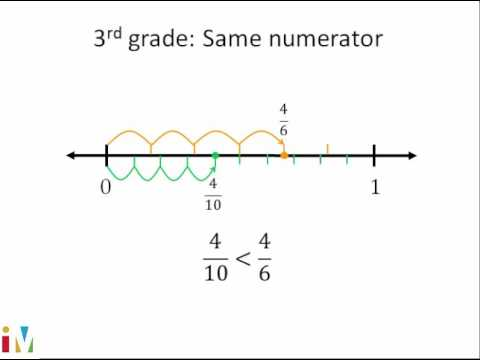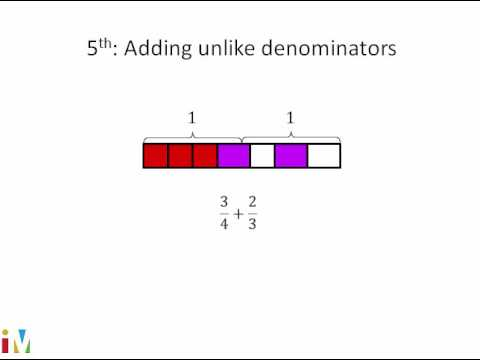Video

# Equivalent fractions visually (Full video)

Description: Sal uses number lines and fraction models to show equivalent fractions. Created by Sal Khan. So what I want you to do is pause this video and think about what fraction the red part represents in each of these shapes. So in this pie right over here, we have 5 equal sections. Now over here, we have 10 equal sections, and 2 of them are shaded in.

### Other videos you might be interested in### Comparing Fractions

#### Illustrative Mathematics